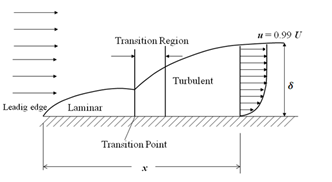Boundary Layer Characteristics ~ MECHTECH GURU

# Boundary Layer Characteristics

The concept of boundary layer was first introduced by a German scientist, Ludwig Prandtl, in the year 1904. Although, the complete descriptions of motion of a viscous fluid were known through Navier-Stokes equations, the mathematical difficulties in solving these equations prohibited the theoretical analysis of viscous flow. Prandtl suggested that the viscous flows can be analyzed by dividing the flow into two regions; one close to the solid boundaries and other covering the rest of the flow. Boundary layer is the regions close to the solid boundary where the effects of viscosity are experienced by the flow. In the regions outside the boundary layer, the effect of viscosity is negligible and the fluid is treated as inviscid. So, the boundary layer is a buffer region between the wall below and the inviscid free-stream above. This approach allows the complete solution of viscous fluid flows which would have been impossible through Navier-Stokes equation. The qualitative picture of the boundary-layer growth over a flat plate is shown in Fig.Representation of boundary layer on a flat plate

A laminar boundary layer is initiated at the leading edge of the plate for a short distance and extends to downstream. The transition occurs over a region, after certain length in the downstream followed by fully turbulent boundary layers. For common calculation purposes, the transition is usually considered to occur at a distance where the Reynolds number is about 500,000. With air at standard conditions, moving at a velocity of 30m/s, the transition is expected to occur at a distance of about 250mm. A typical boundary layer flow is characterized by certain parameters as given below;

## Boundary layer thickness:

It is known that no-slip conditions have to be satisfied at the solid surface: the fluid must attain the zero velocity at the wall. Subsequently, above the wall, the effect of viscosity tends to reduce and the fluid within this layer will try to approach the free stream velocity. Thus, there is a velocity gradient that develops within the fluid layers inside the small regions near to solid surface. The boundary layer thickness is defined as the distance from the surface to a point where the velocity is reaches 99% of the free stream velocity. Thus, the velocity profile merges smoothly and asymptotically into the free stream as shown in Fig.

(a) Boundary layer thickness
(b) Free stream flow (no viscosity)
(c) Concepts of displacement thickness
Previous
Next Post »